•• OpenStax
• OpenStax
$$\newcommand{\vecs}{\overset { \rightharpoonup} {\mathbf{#1}} }$$ $$\newcommand{\vecd}{\overset{-\!-\!\rightharpoonup}{\vphantom{a}\smash {#1}}}$$$$\newcommand{\id}{\mathrm{id}}$$ $$\newcommand{\Span}{\mathrm{span}}$$ $$\newcommand{\kernel}{\mathrm{null}\,}$$ $$\newcommand{\range}{\mathrm{range}\,}$$ $$\newcommand{\RealPart}{\mathrm{Re}}$$ $$\newcommand{\ImaginaryPart}{\mathrm{Im}}$$ $$\newcommand{\Argument}{\mathrm{Arg}}$$ $$\newcommand{\norm}{\| #1 \|}$$ $$\newcommand{\inner}{\langle #1, #2 \rangle}$$ $$\newcommand{\Span}{\mathrm{span}}$$ $$\newcommand{\id}{\mathrm{id}}$$ $$\newcommand{\Span}{\mathrm{span}}$$ $$\newcommand{\kernel}{\mathrm{null}\,}$$ $$\newcommand{\range}{\mathrm{range}\,}$$ $$\newcommand{\RealPart}{\mathrm{Re}}$$ $$\newcommand{\ImaginaryPart}{\mathrm{Im}}$$ $$\newcommand{\Argument}{\mathrm{Arg}}$$ $$\newcommand{\norm}{\| #1 \|}$$ $$\newcommand{\inner}{\langle #1, #2 \rangle}$$ $$\newcommand{\Span}{\mathrm{span}}$$$$\newcommand{\AA}{\unicode[.8,0]{x212B}}$$

##### Learning Objectives

By the end of this section, you will be able to:

• Use the discriminant to predict the number of solutions of a quadratic equation
• Identify the most appropriate method to use to solve a quadratic equation
##### Be Prepared

Before you get started, take this readiness quiz.

1. Simplify: $$\frac{−20−5}{10}$$.
If you missed this problem, review [link].
2. Simplify: $$4+\sqrt{121}$$.
If you missed this problem, review [link].
3. Simplify: $$\sqrt{128}$$.
If you missed this problem, review [link].

When we solved quadratic equations in the last section by completing the square, we took the same steps every time. By the end of the exercise set, you may have been wondering ‘isn’t there an easier way to do this?’ The answer is ‘yes.’ In this section, we will derive and use a formula to find the solution of a quadratic equation.

We have already seen how to solve a formula for a specific variable ‘in general’ so that we would do the algebraic steps only once and then use the new formula to find the value of the specific variable. Now, we will go through the steps of completing the square in general to solve a quadratic equation for x. It may be helpful to look at one of the examples at the end of the last section where we solved an equation of the form$$ax^2+bx+c=0$$ as you read through the algebraic steps below, so you see them with numbers as well as ‘in general.’

 We start with the standard form of a quadratic equation and solve it for x by completing the square. $$ax^2+bx+c=0$$ Isolate the variable terms on one side. $$ax^2+bx=−c$$ Make leading coefficient 1, by dividing by a. $$\frac{ax^2}{a}+\frac{b}{a}x=−\frac{c}{a}$$ Simplify. $$x^2+\frac{b}{a}x=−\frac{c}{a}$$ To complete the square, find$$(\frac{1}{2}·\frac{b}{a})^2$$ and add it to both sides of the equation.$$(\frac{1}{2}\frac{b}{a})^2=\frac{b^2}{4a^2}$$ $$x^2+\frac{b}{a}x+\frac{b^2}{4a^2}=−\frac{c}{a}+\frac{b^2}{4a^2}$$ The left side is a perfect square, factor it. $$(x+\frac{b}{2a})^2=−\frac{c}{a}+\frac{b^2}{4a^2}$$ Find the common denominator of the right side and writeequivalent fractions with the common denominator. $$(x+\frac{b}{2a})^2=−\frac{c·4a}{a·4a}+\frac{b^2}{4a^2}$$ Simplify. $$(x+\frac{b}{2a})^2=\frac{b^2}{4a^2}−\frac{4ac}{4a^2}$$ Combine to one fraction. $$(x+\frac{b}{2a})^2=\frac{b^2−4ac}{4a^2}$$ Use the square root property. $$(x+\frac{b}{2a})=\pm\sqrt{\frac{b^2−4ac}{4a^2}}$$ Simplify. $$(x+\frac{b}{2a})=\pm\frac{\sqrt{b^2−4ac}}{2a}$$ Add $$−\frac{b}{2a}$$to both sides of the equation. $$x=−\frac{b}{2a}\pm\frac{\sqrt{b^2−4ac}}{2a}$$ Combine the terms on the right side. $$x=\frac{−b\pm\sqrt{b^2−4ac}}{2a}$$

This last equation is the Quadratic Formula.

The solutions to a quadratic equation of the form $$ax^2+bx+c=0$$, $$a\ge 0$$ are given by the formula:

$$x=\frac{−b\pm\sqrt{b^2−4ac}}{2a}$$

To use the Quadratic Formula, we substitute the values of a, b, and c into the expression on the right side of the formula. Then, we do all the math to simplify the expression. The result gives the solution(s) to the quadratic equation.

##### Example $$\PageIndex{1}$$

Solve $$2x^2+9x−5=0$$ by using the Quadratic Formula.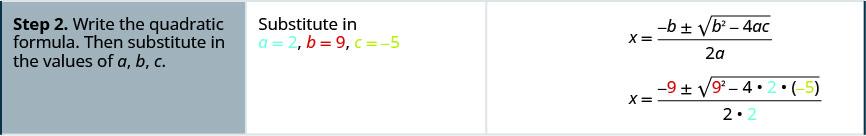##### Example $$\PageIndex{2}$$

Solve $$3y^2−5y+2=0$$ by using the Quadratic Formula.

$$y=\frac{2}{3}$$, $$y=1$$

##### Example $$\PageIndex{3}$$

Solve $$4z^2+2z−6=0$$ by using the Quadratic Formula.

$$z=−\frac{3}{2}$$, $$z=1$$ ​​​​​

1. Write the Quadratic Formula in standard form. Identify the aa, bb, and cc values.
2. Write the Quadratic Formula. Then substitute in the values of a, b, and c.
3. Simplify.
4. Check the solutions.

If you say the formula as you write it in each problem, you’ll have it memorized in no time. And remember, the Quadratic Formula is an equation. Be sure you start with ‘$$x=$$’.

##### Example $$\PageIndex{4}$$

Solve $$x^2−6x+5=0$$ by using the Quadratic Formula.This equation is in standard form.Identify the a, b, c values.Write the Quadratic Formula.Then substitute in the values of a, b, c.Simplify.Rewrite to show two solutions.Simplify.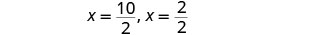Check.##### Example $$\PageIndex{5}$$

Solve $$a^2−2a−15=0$$ by using the Quadratic Formula.

$$a=−3$$, $$a=5$$

##### Example $$\PageIndex{6}$$

Solve $$b^2+10b+24=0$$ by using the Quadratic Formula.

$$b=−6$$, $$b=−4$$

When we solved quadratic equations by using the Square Root Property, we sometimes got answers that had radicals. That can happen, too, when using the Quadratic Formula. If we get a radical as a solution, the final answer must have the radical in its simplified form.

##### Example $$\PageIndex{7}$$

Solve $$4y^2−5y−3=0$$ by using the Quadratic Formula.

We can use the Quadratic Formula to solve for the variable in a quadratic equation, whether or not it is named ‘x’.This equation is in standard form.Identify the a, b, c values.Write the Quadratic Formula.Then substitute in the values of a, b, c.Simplify.Rewrite to show two solutions.Check. We leave the check to you.
##### Example $$\PageIndex{8}$$

Solve $$2p^2+8p+5=0$$ by using the Quadratic Formula.

$$p=\frac{−4\pm\sqrt{6}}{2}$$

##### Example $$\PageIndex{9}$$

Solve $$5q^2−11q+3=0$$ by using the Quadratic Formula.

$$q=\frac{11\pm\sqrt{61}}{10}$$

##### Example $$\PageIndex{10}$$

Solve $$2x^2+10x+11=0$$ by using the Quadratic Formula.This equation is in standard form.Identify the a, b, c values.Write the Quadratic Formula.Then substitute in the values of a, b, c.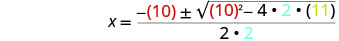Simplify.Simplify the radical.Factor out the common factor in the numerator.Remove the common factors.Rewrite to show two solutions.Check. We leave the check to you.
##### Example $$\PageIndex{11}$$

Solve $$3m^2+12m+7=0$$ by using the Quadratic Formula.

$$m=\frac{−6\pm\sqrt{15}}{3}$$

##### Example $$\PageIndex{12}$$

Solve $$5n^2+4n−4=0$$ by using the Quadratic Formula.

$$n=\frac{−2\pm2\sqrt{6}}{5}$$

We cannot take the square root of a negative number. So, when we substitute a, b, and c into the Quadratic Formula, if the quantity inside the radical is negative, the quadratic equation has no real solution. We will see this in the next example.

##### Example $$\PageIndex{13}$$

Solve $$3p^2+2p+9=0$$ by using the Quadratic Formula.

 This equation is in standard form.Identify the a, b, c values.Write the Quadratic Formula.Then substitute in the values of a, b, c.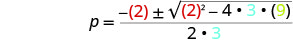Simplify.Simplify the radical.We cannot take the square root of a negative number. There is no real solution.
##### Example $$\PageIndex{14}$$

Solve $$4a^2−3a+8=0$$ by using the Quadratic Formula.

no real solution

##### Exeample $$\PageIndex{15}$$

Solve $$5b^2+2b+4=0$$ by using the Quadratic Formula.

no real solution

The quadratic equations we have solved so far in this section were all written in standard form, $$ax^2+bx+c=0$$. Sometimes, we will need to do some algebra to get the equation into standard form before we can use the Quadratic Formula.

##### Example $$\PageIndex{16}$$

Solve $$x(x+6)+4=0$$ by using the Quadratic Formula.Distribute to get the equation in standard form.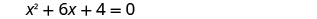This equation is now in standard form.Identify the a, b, c values.Write the Quadratic Formula.Then substitute in the values of a, b, c.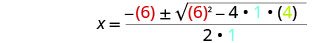Simplify.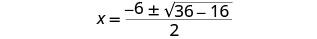Simplify inside the radical.Simplify the radical.Factor out the common factor in the numerator.Remove the common factors.Rewrite to show two solutions.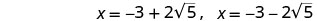Check. We leave the check to you.
##### Example $$\PageIndex{17}$$

Solve $$x(x+2)−5=0$$ by using the Quadratic Formula.

$$x=−1\pm\sqrt{6}$$

##### Example $$\PageIndex{18}$$

Solve $$y(3y−1)−2=0$$ by using the Quadratic Formula.

$$y=−\frac{2}{3}$$, $$y=1$$

When we solved linear equations, if an equation had too many fractions we ‘cleared the fractions’ by multiplying both sides of the equation by the LCD. This gave us an equivalent equation—without fractions—to solve. We can use the same strategy with quadratic equations.

##### Example $$\PageIndex{19}$$

Solve $$\frac{1}{2}u^2+\frac{2}{3}u=\frac{1}{3}$$ by using the Quadratic Formula.Multiply both sides by the LCD, 6, to clear the fractions.Multiply.Subtract 2 to get the equation in standard form.Identify the a, b, c values.Write the Quadratic Formula.Then substitute in the values of a, b, c.Simplify.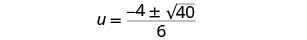Simplify the radical.Factor out the common factor in the numerator.Remove the common factors.Rewrite to show two solutions.Check. We leave the check to you.
##### Example $$\PageIndex{20}$$

Solve $$\frac{1}{4}c^2−\frac{1}{3}c=\frac{1}{12}$$ by using the Quadratic Formula.

$$c=\frac{2\pm\sqrt{7}}{3}$$

##### Example $$\PageIndex{21}$$

Solve $$\frac{1}{9}d^2−\frac{1}{2}d=−\frac{1}{2}$$ by using the Quadratic Formula.

$$d=\frac{3}{2}$$, $$d=3$$

Think about the equation $$(x−3)^2=0$$. We know from the Zero Products Principle that this equation has only one solution: $$x=3$$.

We will see in the next example how using the Quadratic Formula to solve an equation with a perfect square also gives just one solution.

##### Example $$\PageIndex{22}$$

Solve $$4x^2−20x=−25$$ by using the Quadratic Formula.Add 25 to get the equation in standard form.Identify the a, b, c values.Write the Quadratic Formula.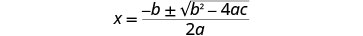Then substitute in the values of a, b, c.Simplify.Simplify the radical.Simplify the fraction.Check. We leave the check to you.

Did you recognize that $$4x^2−20x+25$$ is a perfect square?

##### Example $$\PageIndex{23}$$

Solve $$r^2+10r+25=0$$ by using the Quadratic Formula.

$$r=−5$$​​​​​​

##### Example $$\PageIndex{24}$$

Solve $$25t^2−40t=−16$$ by using the Quadratic Formula.

$$t=\frac{4}{5}$$

## Use the Discriminant to Predict the Number of Solutions of a Quadratic Equation

When we solved the quadratic equations in the previous examples, sometimes we got two solutions, sometimes one solution, sometimes no real solutions. Is there a way to predict the number of solutions to a quadratic equation without actually solving the equation?

Yes, the quantity inside the radical of the Quadratic Formula makes it easy for us to determine the number of solutions. This quantity is called the discriminant.

##### Definition: DISCRIMINANT

In the Quadratic Formula$$x=\frac{−b\pm\sqrt{b^2−4ac}}{2a}$$, the quantity $$b^2−4ac$$ is called the discriminant.

Let’s look at the discriminant of the equations in Example, Example, and Example, and the number of solutions to those quadratic equations.

Quadratic Equation (in standard form) Discriminant $$b^2−4ac$$ Sign of the Discriminant Number of real solution
Example $$2x^2+9x−5=0$$ $$9^2−4·2(−5)=121$$ + 2
Example $$4x^2−20x+25=0$$ $$(−20)^2−4·4·25=0$$ 0 1
Example $$3p^2+2p+9=0$$ $$2^2−4·3·9=−104$$ 0

When the discriminant is positive $$x=\frac{−b\pm\sqrt{+}}{2a}$$ the quadratic equation has two solutions.

When the discriminant is zero $$x=\frac{−b\pm\sqrt{0}}{2a}$$ the quadratic equation has one solution.

When the discriminant is negative $$x=\frac{−b\pm\sqrt{−}}{2a}$$ the quadratic equation has no real solutions.

##### Definition:USE THE DISCRIMINANT, $$b^2−4ac$$, TO DETERMINE THE NUMBER OF SolutionS OF A QUADRATIC EQUATION

For a quadratic equation of the form $$ax^2+bx+c=0$$, $$a \ge 0$$,

• if $$b^2−4ac>0$$, the equation has two solutions.
• if $$b^2−4ac=0$$, the equation has one solution.
• if $$b^2−4ac<0$$, the equation has no real solutions.
##### Example $$\PageIndex{25}$$

Determine the number of solutions to each quadratic equation:

1. $$2v^2−3v+6=0$$
2. $$3x^2+7x−9=0$$
3. $$5n^2+n+4=0$$
4. $$9y^2−6y+1=0$$

1.

 $$2v^2−3v+6=0$$ The equation is in standard form, identify a, b, c. $$a=2$$, $$b=−3$$, $$c=6$$ Write the discriminant. $$b^2−4ac$$ Substitute in the values of a, b, c. $$(3)^2−4·2·6$$ Simplify. $$9−48$$ $$−39$$ Because the discriminant is negative, there are no real solutions to the equation.

2.

 $$3x^2+7x−9=0$$ The equation is in standard form, identify a, b, c. $$a=3$$, $$b=7$$, $$c=−9$$ Write the discriminant. $$b^2−4ac$$ Substitute in the values of a, b, c. $$(7)^2−4·3·(−9)$$ Simplify. $$49+108$$ $$157$$ Because the discriminant is positive, there are two solutions to the equation.

3.

 $$5n^2+n+4=0$$ The equation is in standard form, identify a, b, c. $$a=5$$, $$b=1$$, $$c=4$$ Write the discriminant. $$b^2−4ac$$ Substitute in the values of a, b, c. $$(1)^2−4·5·4$$ Simplify. $$1−80$$ $$−79$$ Because the discriminant is negative, there are no real solutions to the equation.

4.

 $$9y^2−6y+1=0$$ The equation is in standard form, identify a, b, c. $$a=9$$, $$b=−6$$, $$c=1$$ Write the discriminant. $$b^2−4ac$$ Substitute in the values of a, b, c. $$(−6)^2−4·9·1$$ Simplify. $$36−36$$ $$0$$ Because the discriminant is 0, there is one solution to the equation.
##### Example $$\PageIndex{26}$$

Determine the number of solutions to each quadratic equation:

1. $$8m^2−3m+6=0$$
2. $$5z^2+6z−2=0$$
3. $$9w^2+24w+16=0$$
4. $$9u^2−2u+4=0$$
1. no real solutions
2. 2
3. 1
4. no real solutions
##### Example $$\PageIndex{27}$$

​​​​​​​Determine the number of solutions to each quadratic equation:

1. $$b^2+7b−13=0$$
2. $$5a^2−6a+10=0$$
3. $$4r^2−20r+25=0$$
4. $$7t^2−11t+3=0$$
1. 2
2. no real solutions
3. 1
4. 2

## Identify the Most Appropriate Method to Use to Solve a Quadratic Equation

We have used four methods to solve quadratic equations:

• Factoring
• Square Root Property
• Completing the Square

You can solve any quadratic equation by using the Quadratic Formula, but that is not always the easiest method to use.

##### Definition: IDENTIFY THE MOST APPROPRIATE METHOD TO SOLVE A QUADRATIC EQUATION.
1. Try Factoring first. If the quadratic factors easily, this method is very quick.
2. Try the Square Root Property next. If the equation fits the form $$ax^2=k$$ or $$a(x−h)^2=k$$, it can easily be solved by using the Square Root Property.

​​​​​​What about the method of completing the square? Most people find that method cumbersome and prefer not to use it. We needed to include it in this chapter because we completed the square in general to derive the Quadratic Formula. You will also use the process of completing the square in other areas of algebra.

##### Example $$\PageIndex{28}$$

Identify the most appropriate method to use to solve each quadratic equation:

1. $$5z^2=17$$
2. $$4x^2−12x+9=0$$
3. $$8u^2+6u=11$$

1. $$5z^2=17$$

Since the equation is in the $$ax^2=k$$, the most appropriate method is to use the Square Root Property.

2. $$4x^2−12x+9=0$$

We recognize that the left side of the equation is a perfect square trinomial, and so Factoring will be the most appropriate method.

3. $$8u^2+6u=11$$

Put the equation in standard form. $$8u^2+6u−11=0$$

While our first thought may be to try Factoring, thinking about all the possibilities for trial and error leads us to choose the Quadratic Formula as the most appropriate method.

##### Example $$\PageIndex{29}$$

Identify the most appropriate method to use to solve each quadratic equation:

1. $$x^2+6x+8=0$$
2. $$(n−3)^2=16$$
3. $$5p^2−6p=9$$
1. factor
2. Square Root Property
##### Example $$\PageIndex{30}$$

Identify the most appropriate method to use to solve each quadratic equation:

1. $$8a^2+3a−9=0$$
2. $$4b^2+4b+1=0$$
3. $$5c2=125$$
2. factoring
3. Square Root Property

​​​​​​​Access these online resources for additional instruction and practice with using the Quadratic Formula:

## Key Concepts

• Quadratic Formula The solutions to a quadratic equation of the form $$ax^2+bx+c=0$$, $$a \ge 0$$ are given by the formula:

$$x=\frac{−b\pm\sqrt{b^2−4ac}}{2a}$$

1. Write the quadratic formula in standard form. Identify the a, b, c values.
2. Write the quadratic formula. Then substitute in the values of a, b, c.
3. Simplify.
4. Check the solutions.
• Using the Discriminant, $$b^2−4ac$$, to Determine the Number of Solutions of a Quadratic Equation
For a quadratic equation of the form $$ax^2+bx+c=0$$, $$a \ge 0$$ ​​,
• if $$b^2−4ac>0$$, the equation has 2 solutions.
• if $$b^2−4ac=0$$, the equation has 1 solution.
• if $$b^2−4ac<0$$, the equation has no real solutions.
• To identify the most appropriate method to solve a quadratic equation:
1. Try Factoring first. If the quadratic factors easily this method is very quick.
2. Try the Square Root Property next. If the equation fits the form $$ax^2=k$$ or $$a(x−h)^2=k$$, it can easily be solved by using the Square Root Property.
3. Use the Quadratic Formula. Any other quadratic equation is best solved by using the Quadratic Formula.

## Glossary

discriminant
In the Quadratic Formula,$$x=\frac{−b\pm\sqrt{b^2−4ac}}{2a}$$ the quantity $$b^2−4ac$$ is called the discriminant.